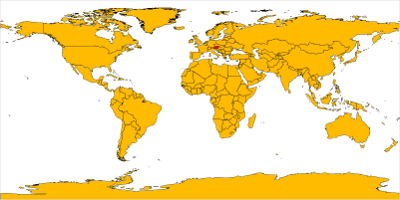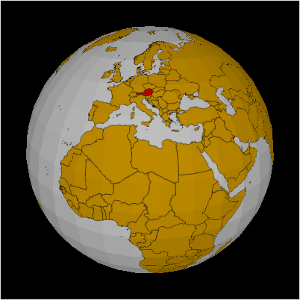## projecting GIS Data on a sphere using Processing

i have used processing to access map materials from geoserver. but i have noticed that the earth is not flat and that processing is making 3d animations really easy :-)

so i have coded a rotating sphere and project the GIS data from geoserver on the surface

flat map from my last sketchspherical map from the new sketchThe code accesses the geoserver only in the setup method yet. If the data that is displayed varies over the time, update it in the draw method.

``````PImage world;

void setup() {
size(400,400, P3D);
frameRate( 25 );
//smooth();
world = getWMS( "http://localhost:8080/geoserver/wms", "topp:TM_WORLD_BORDERS-0", -180, -90, 180, 90, 1600, 800 );
}

float a = 0;

void draw() {
background( 0 );
lights();
noStroke();
fill(128);
translate( width/2, height/2);

pushMatrix();
rotateY( a );
a+= 0.01;
makeSphere( 150, 10, world );

popMatrix();
}

PImage getWMS( String url, String layer, float minlon, float minlat, float maxlon, float maxlat, int width, int height ) {
PImage res = loadImage( url + "?request=GetMap&layers=" + layer +"&bbox="+minlon+","+minlat+","+maxlon+","+maxlat+"&format=image/png&width="+width+"&height="+height, "png");
return res;
}

public void makeSphere( int R, int step, PImage tex ) {
beginShape(TRIANGLE_STRIP);
texture( tex );
for( int i = 0; i < 90; i+=step ) {
float sini = sin( radians( i ));
float cosi = cos( radians( i ));
float sinip = sin( radians( i + step ));
float cosip = cos( radians( i + step ));

for( int j = 0; j < 360; j+=step ) {
float sinj = sin( radians( j ));
float cosj = cos( radians( j ));
float sinjp = sin( radians( j + step ));
float cosjp = cos( radians( j + step ));

// Upper hemisphere
vertex( R * cosj * sini, R * -cosi, R * sinj * sini, // x, y, z
tex.width-j * tex.width / 360, i * tex.height / 180 // u, v
);

vertex( R * cosjp * sini, R * -cosi, R * sinjp * sini,
tex.width-(j + step ) * tex.width / 360, i * tex.height / 180
);

vertex( R * cosj * sinip, R * -cosip, R * sinj * sinip,
tex.width-j * tex.width / 360, (i + step ) * tex.height / 180
);

vertex( R * cosjp * sinip, R * -cosip, R * sinjp * sinip,
tex.width-(j + step ) * tex.width / 360, (i + step ) * tex.height / 180
);

// Lower hemisphere
vertex( R * cosj * sini, R * cosi, R * sinj * sini,
tex.width-j * tex.width / 360, tex.height - i * tex.height / 180
);

vertex( R * cosjp * sini, R * cosi, R * sinjp * sini,
tex.width-(j + step ) * tex.width / 360, tex.height - i * tex.height / 180
);

vertex( R * cosj * sinip, R * cosip, R * sinj * sinip,
tex.width-j * tex.width / 360, tex.height - (i+step) * tex.height / 180
);

vertex( R * cosjp * sinip, R * cosip, R * sinjp * sinip,
tex.width-(j+step) * tex.width / 360, tex.height - (i+step) * tex.height / 180
);
}
}
endShape();
}
``````

Leave a response

1. thanks.. Nice good article.

2. when i try to use your code i get a NullPointerException error, do you know what is causing this? It seems to be coming from the hemisphere creation.

3. hi j,

make sure that the processing code can load the texture image from the mapping server. If the texture is not loadable then the vertext command dies with a NullPointerException while trying to access width and height of the texture.

4. betclic Merci pour les information et bonne continuation je reviendrai merci

5. Good day-

We are working on an educational exhibit about Armenian culture and would like to use a high-rez version of the country borders mapped onto the sphere on your web site.

Is it available?

Thank you,

Ed Malouf

6. @Ed Malouf